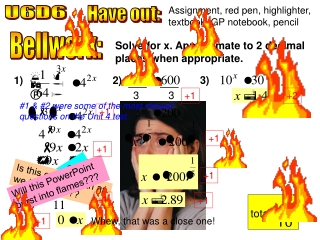Download Presentationtotal:

# total:

Télécharger la présentation## total:

- - - - - - - - - - - - - - - - - - - - - - - - - - - E N D - - - - - - - - - - - - - - - - - - - - - - - - - - -
##### Presentation Transcript

1. total: Assignment, red pen, highlighter, textbook, GP notebook, pencil U6D6 Have out: Bellwork: Solve for x. Approximate to 2 decimal places when appropriate. 3) 1) 2) 3 3 +1 +2 #1 & #2 were some of the most missed questions on the Unit 4 test. +1 +1 +1 +1 Will this PowerPoint burst into flames??? Is this end of math as we know it???? No solution??? +1 +1 +1 Whew, that was a close one!

2. y 10 x -10 10 -10 Copy onto your worksheet: Introduction to Logarithms Sketch y = f(x) for f(x) = 10x. We know the graph of the inverse function f–1(x) will be the __________ of y = f(x) across the line of _________. y = x reflection reflection f–1(x) f(x) = 10x 1 10 Sketch y = f–1(x) and y = x.

3. y 10 x -10 10 -10 What is f–1(x)? What undoes the exponential function? y = x y logarithm The ___________ function is the inverse (or undoes) the exponential function. f–1(x) f(x) = 10x Notation: f–1(x) = log10x x base The “10” is called the _____ of the logarithm, just like it is the base in the exponential function. common “10” is also called the _________ logarithm, so we omit the 10. log10x log x Therefore _________ implies _________. (This is similar to the unwritten “one” for coefficients or exponents.)

4. When we use the logarithmic function, we are looking for an _________. exponent 10 to what power is equal to 100? log 100 means “_____________________________” 2 log 100 = ____ Practice: 3 –1 0 4 –3 –2

5. y 5 4 3 2 1 x 1 2 3 4 5 -5 -4 -3 -2 -1 -1 -2 -3 -4 -5 For each function, sketch y = f(x), y = f–1(x), and y = x. Determine f–1(x). f(x) = 2x y = x a) f(x) = 2x (–∞, ∞) Domain: _______ f–1(x) (0, ∞) Range: _______ 1 2 4 log2x f–1(x) = _______ f–1(x) = log2(x) f(x) = 2x (0, ∞) Domain: _______ (–∞, ∞) Range: _______ same base

6. y 5 4 3 2 1 x 1 2 3 4 5 -5 -4 -3 -2 -1 -1 -2 -3 -4 -5 For each function, sketch y = f(x), y = f–1(x), and y = x. Determine f–1(x). f(x) = 5x y = x b) f(x) = 5x (–∞, ∞) Domain: _______ (0, ∞) Range: _______ 1 f–1(x) 5 log5x f–1(x) = _______ (0, ∞) Domain: _______ (–∞, ∞) Range: _______ f–1(x) = log5(x) f(x) = 5x Given b > 0, f(x) = logbx has domain ____ and range ______. (0, ∞) (–∞, ∞) same base

7. Practice: Simplify these logarithmic expressions. Recall: “the base to what power is…?” 2 4 3 5 –2 –3 –2 –4 –3 –3 Click here

8. Finish today's assignment: Log Mixed Practice & CC 60, 61, 64, 66, 68